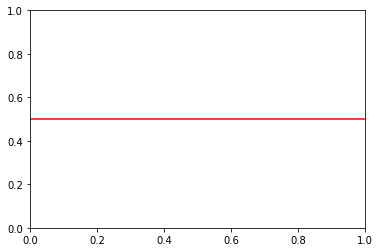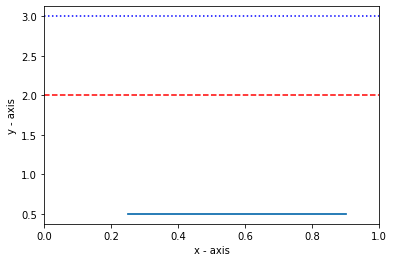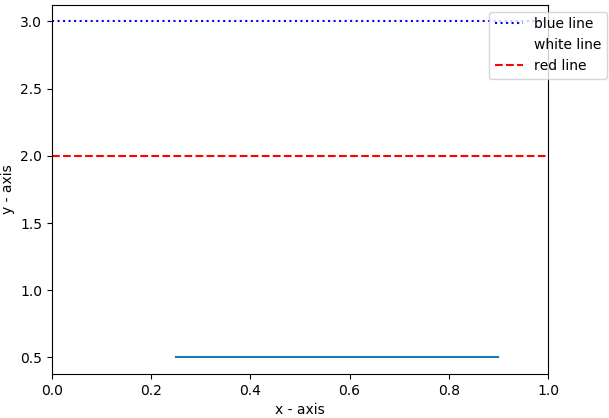# Plot a Horizontal line in Matplotlib

Matplotlib is a popular python library used for plotting, It provides an object-oriented API to render GUI plots

Plotting a horizontal line is fairly simple, Using axhline()

The axhline() function in pyplot module of matplotlib library is used to add a horizontal line across the axis.

Syntax: matplotlib.pyplot.axhline(y, color, xmin, xmax, linestyle)

Parameters:

• y: Position on Y axis to plot  the line, It accepts integers.
• xmin and xmax: scalar, optional, default: 0/1.  It plots the line in the given range
• color: color for the line, It accepts  a string. eg ‘r’ or ‘b’ .
• linestyle: Specifies the type of line, It accepts a string. eg ‘-‘, ‘–‘, ‘-.’, ‘:’, ‘None’, ‘ ‘, ”, ‘solid’, ‘dashed’, ‘dashdot’, ‘dotted’

## Python3

 `# importing library ` `import` `matplotlib.pyplot as plt ` ` `  `# specifying horizontal line type ` `plt.axhline(y ``=` `0.5``, color ``=` `'r'``, linestyle ``=` `'-'``) ` ` `  `# rendering the plot ` `plt.show()`

Output:### Plotting multiple horizontal lines

To plot multiple horizontal lines, use the axhline() method multiple times.

## Python

 `# importing the module ` `import` `matplotlib.pyplot as plt ` ` `  `# plotting line within the given range ` `plt.axhline(y ``=` `.``5``, xmin ``=` `0.25``, xmax ``=` `0.9``) ` ` `  `# line colour is blue ` `plt.axhline(y ``=` `3``, color ``=` `'b'``, linestyle ``=` `':'``) ` ` `  `# line colour is white ` `plt.axhline(y ``=` `1``, color ``=` `'w'``, linestyle ``=` `'--'``) ` ` `  `# line colour is red ` `plt.axhline(y ``=` `2``, color ``=` `'r'``, linestyle ``=` `'dashed'``)     ` `   `  `# adding axis labels     ` `plt.xlabel(``'x - axis'``) ` `plt.ylabel(``'y - axis'``) ` ` `  `# displaying the plot ` `plt.show()`

Output:The legend can be added using the legend() function.

## Python3

 `# importing the module ` `import` `matplotlib.pyplot as plt ` ` `  `# plotting line within the given range ` `plt.axhline(y ``=` `.``5``, xmin ``=` `0.25``, xmax ``=` `0.9``) ` ` `  `# line colour is blue ` `plt.axhline(y ``=` `3``, color ``=` `'b'``, linestyle ``=` `':'``, label ``=` `"blue line"``) ` ` `  `# line colour is white ` `plt.axhline(y ``=` `1``, color ``=` `'w'``, linestyle ``=` `'--'``, label ``=` `"white line"``) ` ` `  `# line colour is red ` `plt.axhline(y ``=` `2``, color ``=` `'r'``, linestyle ``=` `'dashed'``, label ``=` `"red line"``)     ` `   `  `# adding axis labels     ` `plt.xlabel(``'x - axis'``) ` `plt.ylabel(``'y - axis'``) ` ` `  `# plotting the legend ` `plt.legend(bbox_to_anchor ``=` `(``1.0``, ``1``), loc ``=` `'upper center'``) ` ` `  `# displaying the plot ` `plt.show()`

Output:Whether you're preparing for your first job interview or aiming to upskill in this ever-evolving tech landscape, GeeksforGeeks Courses are your key to success. We provide top-quality content at affordable prices, all geared towards accelerating your growth in a time-bound manner. Join the millions we've already empowered, and we're here to do the same for you. Don't miss out - check it out now!

Previous
Next